# 廣義量詞系列：量詞的博奕性質

## 1. 引言

「博奕論」(Game Theory)是在當代發展起來的應用數學學科，在多個學科領域中都有應用。一場「博奕」 (Game)包含以下因素：一組「對奕者」(Player)，這些「對奕者」所能採取的「行動」(Move)或「策略」 (Strategy)，以及各種「行動」組合所引致的「賽果」(Payoff)，此一「賽果」可以採取勝負或分數的形式。

## 2. 公式博奕

### 2.1.1 基本形式

「公式博奕」(Formula Game)是指以邏輯公式為博奕對象的博奕，最簡單的「公式博奕」以「命題邏 輯」公式為博奕對象，這種博奕在「計算複雜性理論」中也有所研究。本節要介紹的博奕是以「謂詞邏輯」公 式作為博奕對象，Hintikka、Kulas、Sandu等人把這種博奕應用於經典量詞(即"∀"和"∃")的語義 解釋，從而開創了「博奕論語義學」(Game-Theoretic Semantics)，本小節介紹這方面的基礎知識。

1

2
~S0

3
S1 ∧ S2
F選擇i = 1或2，然後繼續進 行G(Si, M)。
4
S1 ∨ S2
V選擇i = 1或2，然後繼續進 行G(Si, M)。
5
∀x (S0)
F在M的論域U中選擇一個個體a，然後 繼續進行G(S0[a/x], M)。
6
∃x (S0)
V在M的論域U中選擇一個個體a，然後繼 續進行G(S0[a/x], M)。

S1 = ∀x∃y ((BOY(x) ∧ GIRL(y)) → ~LOVE(x, y))     (1)

 U = {a, b, c, d} BOY = {a, b}, GIRL = {c, d}, LOVE = {(a, a), (a, c), (b, d), (c, b), (c, c)}

S1 = ∀x∃y (~(BOY(x) ∧ GIRL(y)) ∨ ~LOVE(x, y))     (2)

S2 = ∃y (~(BOY(a) ∧ GIRL(y)) ∨ ~LOVE(a, y))     (3)

S3 = ~(BOY(a) ∧ GIRL(d)) ∨ ~LOVE(a, d)     (4)

S4 = ~(BOY(c) ∧ GIRL(c)) ∨ ~LOVE(c, c)     (5)

### 2.1.2 變化形式

x ∈ U (x ∈ A ⇒ x ∈ B)     (6)
x ∈ A (x ∈ B)     (7)

 5' ∀x ∈ A (S0) F在集合A中選 擇一個個體a，然後繼續進行G(S0[a/x], M)。 6' ∃x ∈ A (S0) V在集合A中選 擇一個個體a，然後繼續進行G(S0[a/x], M)。

Hintikka等人開創的「博奕論語義學」，除了用來解釋謂詞邏輯公式的語義外，也用來解釋自然語言量化句的 語義。因此除了表1的規則外，他們也為自然語言的邏輯聯結詞(例如"and"、"or"等)、量詞(例如"every"、 "some"等)設計了相應的博奕規則，而且他們的規則是建基於自然語言的句子形式而非邏輯表達式，下表是有關 "every"和"some"的規則(下表略去了「原子句子」、否定(以"~"表示)、"and"和"or"的規則，這是因為這些規 則跟表1的相應規則大同小異)：

5
"X every A Y"
F在集合A中選擇一個個體x，然後繼續進行G("X x Y", M)。
6
"X some A Y"
V在集合A中選擇一個個體x，然後繼續進行G("X x Y", M)。

### 2.2.1 限定詞

 7 "X at least n A Y" V在集合A中選擇一個子集A'使得|A'| ≥ n ，然後繼續進行G("X every A' Y", M)。 8 "X more than n A Y" V在集合A中選擇一個子集A'使得|A'| > n， 然後繼續進行G("X every A' Y", M)。

A' ⊆ A ∧ |A'| ≥ na ∈ A' (a ∈ B)     (8)

 9 "X exactly n A Y" V在集合A中選擇一個子集A'使得|A'| = n，然 後繼續進行G("X every A' Y and X no (A − A') Y", M)。 10 "X all except n A Y" V在集合A中選擇一個子集A'使得|A'| = n ，然後繼續進行G("X no A' Y and every (A − A') Y", M)。

A' ⊆ A ∧ |A'| = n (∀a ∈ A' (a ∈ B) ∧ ~∃a ∈ A − A' (a ∈ B))     (9)

A' ⊆ B ∧ (A − A') ∩ B = Φ     (10)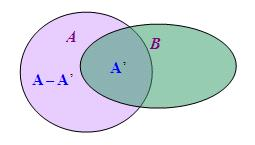11 "X at least q A Y" V在集合A中選擇一個子集A'使得|A'| / |A| ≥ q，然後繼續進行G("X every A' Y", M)。 12 "X more than q A Y" V在集合A中選擇一個子集A'使得|A'| / |A| > q，然後繼續進行G("X every A' Y", M)。 13 "X exactly q A Y" V在集合A中選擇一個子集A'使得|A'| / |A| = q，然後繼續進行G("X every A' Y and X no (A − A') Y", M)。 14 "X all except q A Y" V在集合A中選擇一個子集A'使得|A'| / |A| = q，然後繼續進行G("X no A' Y and every (A − A') Y", M)。

### 2.2.2 結構化量詞

 15 "X at least n more A than B Y" V選擇一個自然數k，然後繼續 進行G("X at least (k + n) A Y and X at most k B Y", M)。 16 "X exactly n more A than B Y" V選擇一個自然數k，然後繼續進 行G("X exactly (k + n) A Y and X exactly k B Y", M)。 17 "X at least n times as many A as B Y" V選擇一個自然數k，然 後繼續進行G("X at least nk A Y and X at most k B Y", M)。 18 "X exactly n times as many A as B Y" V選擇一個自然數k，然 後繼續進行G("X exactly nk A Y and X exactly k B Y", M)。 19 "X at least q more A than B Y" V選擇一個自然數k，然後繼續 進行G("X at least (1 + q)k A Y and X at most k B Y", M)。 20 "X exactly q more A than B Y" V選擇一個自然數k，然後繼續進 行G("X exactly (1 + q)k A Y and X exactly k B Y", M)。 21 "X proportionally more A than B Y" V選擇一個有理數q (0 < q < 1)，然後繼續進行G("X more than q A Y and X at most q B Y", M)。 22 "X at least the same proportion of A as B Y" V選擇一個有理 數q (0 < q < 1)，然後繼續進行G("X at least q A Y and X at most q B Y", M)。 23 "X exactly the same proportion of A as B Y" V選擇一個有理 數q (0 < q < 1)，然後繼續進行G("X exactly q A Y and X exactly q B Y", M)。

at least n more Z A than B

V選擇一個自然數k，然後繼續進行G("at least (k + n) Z A and at most k Z B", M)。

「第二類結構化量詞」全都具有「<1,12>」的類型，以下是這些量詞的博奕規則：

 24 "the same Z A as B" V在集合A和B中各選擇一個子集A'和B'使得 A' = B'，然後繼續進行G("every A' is a Z and no (A − A') is a Z and every B' is a Z and no (B − B') is a Z", M)。 25 "differentw Z A than B" V在集合A和B中各選擇一個 子集A'和B'使得A' ≠ B'，然後繼續進行G("every A' is a Z and no (A − A') is a Z and every B' is a Z and no (B − B') is a Z", M)。 26 "differents Z A than B" V在集合A和B中各選擇一個 子集A'和B'使得A' ∩ B' = Φ，然後繼續進行G("every A' is a Z and no (A − A') is a Z and every B' is a Z and no (B − B') is a Z", M)。 27 "whatever Z A also B" V在集合A和B中各選擇一個子集A'和B'使 得A' ⊆ B'，然後繼續進行G("every A' is a Z and no (A − A') is a Z and every B' is a Z and no (B − B') is a Z", M)。

### 2.2.3 迭代量詞

S5 = All except 2 teachers recommended more girls than boys.     (11)

 U = {a, b, c, d, e, f, g, h, i, j} TEACHER = {g, h, i, j}, BOY = {a, b}, GIRL = {c, d, e, f} RECOMMEND = {(g, a), (g, c), (h, b), (i, b), (i, e), (i, f), (j, a), (j, b), (j, d), (j, e)}

S5 = all except 2 TEACHER RECOMMEND more GIRL than BOY     (12)

S6 = no {g, h} RECOMMEND more GIRL than BOY
and every (TEACHER − {g, h}) RECOMMEND more GIRL than BOY     (13)

S7 = every (TEACHER − {g, h}) RECOMMEND more GIRL than BOY     (14)

S8 = j RECOMMEND more GIRL than BOY     (15)

S8 = j RECOMMEND at least 1 more GIRL than BOY     (16)

S9 = j RECOMMEND at least 2 GIRL and j RECOMMEND at most 1 BOY     (17)

S10 = j RECOMMEND at most 1 BOY     (18)

S10 = j RECOMMEND ~more than 1 BOY     (19)

### 2.2.4 概括量詞與複雜結構化量詞組合

No student is waiting for any student except John for Bill.     (20)

Except for the pair (John, Bill), no pair of students (x, y) is such that x is waiting for y.     (21)

no STUDENT2 except (j, b) WAIT-FOR     (22)

No two boys answered the same questions.     (23)
Every boy knows more girls than some teacher does.     (24)

There is no unordered pair of boys {x, y} such that the same questions are answered by x as answered by y.     (25)
For every boy x there is some teacher y such that more girls are known by x than known by y.     (26)

~some {{x, y}: BOY(x) ∧ BOY(y)} the same QUESTION ANSWERED-BY-x as ANSWERED-BY-y     (27)
every {x: BOY(x)} some {y: TEACHER(y)} more GIRL KNOWN-BY-x than KNOWN-BY-y     (28)

### 2.2.5 分枝量詞與累指量詞

「分枝量詞」與前述「迭代量詞」的區別在於，後者的各個量化短語處於同一條直線上(故又稱「線性量詞」) ，互有統屬關係，處於窄域的量化短語隸屬於處於寬域的量化短語；前者的各個量化短語則處於不同分枝，不 同分枝上的量化短語互相獨立(故又稱「獨立量詞」)，互不隸屬，這裡「A隸屬B」的意思是A的語義所指依賴於 B的語義所指。舉例說，

Every boy loves some girl.     (29)

Two students passed four of the exams.     (30)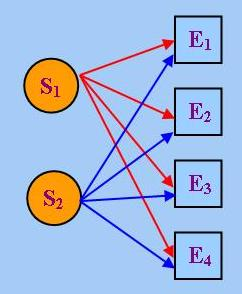exactly 2 {x: STUDENT(x)} x PASS y     (31) exactly 4 {y: EXAM(y)}

 j j PASS y     (32) exactly 4 {y: EXAM(y)}

Some girl loves some boy.     (33)

some GIRL LOVE some BOY     (34)

 some {x: GIRL(x)} x LOVE y     (35) some {y: BOY(y)}

Eight examiners interviewed 100 candidates.     (36)

 exactly 8 {x: EXAMINER(x)} x INTERVIEW some C' and some E' INTERVIEW y     (37) exactly 100 {y: CANDIDATE(y)}

## 3. 結構博奕

### 3.1 基本概念

「結構博奕」(Structure Game)以邏輯結構作為博奕對象，最初由Ehrenfeucht和Fraisse提出。在開 始介紹「結構博奕」前，我們須先引入數理邏輯中「詞匯」(Vocabulary)(亦稱「特徵」Signature)的概念，一 個「邏輯結構」(亦稱「模型」)的「詞匯」就是指描述該結構特性所要用到的各種特定常項、集合、函數或關 係符號的集合。舉例說，在抽象代數學上，整數集與加法運算構成一個「群」(Group)。如果我們把這個群看成 一個邏輯結構，那麼這個結構的「詞匯」便包括一個代表「加法單位元」的常項符號"0"以及一個代表「加法運 算」的二元函數符號"+"。

P(mi ... mj) ⇔ P(f(mi) ... f(mj))     (38)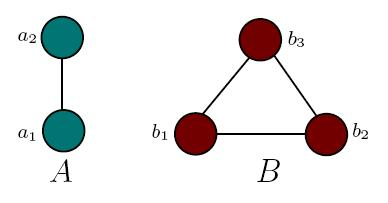EDGE(a2, a1) ⇔ EDGE(f(a2), f(a1))

### 3.2 量詞深度與前束範式

1. Depth(p) = 0，若p為原子公式；
2. Depth(p) = Depth(q)，若p = ~q；
3. Depth(p) = max(Depth(q), Depth(r))，若p = q ∧ r；
4. Depth(p) = max(Depth(q), Depth(r))，若p = q ∨ r；
5. Depth(p) = Depth(q) + 1，若p = ∀x(q(x))；
6. Depth(p) = Depth(q) + 1，若p = ∃x(q(x))；

 Depth(∀x (∃y (~R(x, y)) ∧ ∀z (P(x, z)))) = Depth(∃y (~R(x, y)) ∧ ∀z (P(x, z))) + 1 應用5 = max(Depth(∃y (~R(x, y))), Depth(∀z (P(x, z)))) + 1 應用3 = max(Depth(~R(x, y)) + 1, Depth(P(x, z)) + 1) + 1 應用6和5 = max(Depth(R(x, y)) + 1, Depth(P(x, z)) + 1) + 1 應用2 = max(0 + 1, 0 + 1) + 1 應用1 = max(1, 1) + 1 = 1 + 1 = 2

「前束範式」(Prenex Normal Form)則是一階語句的某種特定形式，我們說一階語句p表現為「前束 範式」當且僅當p由前後兩部分組成，前一部分是一串量詞，稱為「前綴」(Prefix)，後一部分則是不含任何量 詞的公式，稱為「主體」(Matrix)。舉例說，

∀x∃y∃z (q(y) ∨ (r(z) ⇒ p(x)))     (39)

∀x (∃y(q(y)) ∨ (∀z(r(z)) ⇒ p(x)))     (40)

1. ∀x(p) ∧ q ≡ ∀x (p ∧ q)
2. ∀x(p) ∨ q ≡ ∀x (p ∨ q)
3. ∃x(p) ∧ q ≡ ∃x (p ∧ q)
4. ∃x(p) ∨ q ≡ ∃x (p ∨ q)
5. ~∀x(p) ≡ ∃x(~p)
6. ~∃x(p) ≡ ∀x(~p)

 ∀x (∃y(q(y)) ∨ (∀z(r(z)) ⇒ p(x))) ≡ ∀x (∃y(q(y)) ∨ (~∀z(r(z)) ∨ p(x))) 應用"⇒"的定義 ≡ ∀x (∃y(q(y)) ∨ (∃z(~r(z)) ∨ p(x))) 應用5 ≡ ∀x (∃y(q(y)) ∨ ∃z (~r(z) ∨ p(x))) 應用4 ≡ ∀x (∃y (q(y) ∨ ∃z (~r(z) ∨ p(x)))) 應用4 ≡ ∀x (∃y (∃z (~r(z) ∨ p(x)) ∨ q(y))) 應用交 換律 ≡ ∀x (∃y (∃z (~r(z) ∨ p(x) ∨ q(y)))) 應用4和結 合律 ≡ ∀x (∃y (∃z (q(y) ∨ ~r(z) ∨ p(x)))) 應用交換 律 ≡ ∀x∃y∃z (q(y) ∨ (r(z) ⇒ p(x))) 應用結合律和 "⇒"的定義，並略去不必要的括號

### 3.3 基本定理

p = Q1x1Q2x2....Qrxr (p'(x1, x2, ... xr))

~p = Q1'x1Q2'x2.... Qr'xr (~p'(x1, x2, ... xr))

Q1x1Q2x2....Qrxr (p'(x1, x2, ... xr))在M中真     (41)
Q1'x1Q2'x2....Qr'xr (~p'(x1, x2, ... xr))在N中真     (42)

Q2x2....Qrxr (p'(m1, x2, ... xr))在M中真     (43)

Q2'x2....Qr'xr (~p'(n1, x2, ... xr))在N中真     (44)

Qi+1x1....Qrxr (p'(m1, ... mi, xi+1, ... xr))在M中真     (45)
Qi+1'x1....Qr'xr (~p'(n1, ... ni, xi+1, ... xr))在N中真     (46)

p'(m1, ... mr)在M中真     (47)
~p'(n1, ... nr)在N中真     (48)

p'(n1, ... nr)在N中假     (49)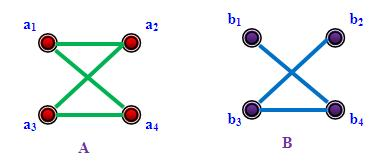p = ∀x∃y∃z (EDGE(x, y) ∧ EDGE(x, z) ∧ y ≠ z)在A中真     (50)
~p = ∃x∀y∀z (~EDGE(x, y) ∨ ~EDGE(x, z) ∨ y = z)在B中真     (51)

∃y∃z (EDGE(a1, y) ∧ EDGE(a1, z) ∧ y ≠ z)在A 中真     (52)
∀y∀z (~EDGE(b1, y) ∨ ~EDGE(b1, z) ∨ y = z)在B中真     (53)

∃z (EDGE(a1, a2) ∧ EDGE(a1, z) ∧ a2 ≠ z)在A中真     (54)
∀z (~EDGE(b1, b4) ∨ ~EDGE(b1, z) ∨ b4 = z)在B中真     (55)

EDGE(a1, a2) ∧ EDGE(a1, a4) ∧ a2 ≠ a4在A中真     (56)
~EDGE(b1, b4) ∨ ~EDGE(b1, b3) ∨ b4 = b3在B中真     (57)

### 3.4 一階不可定義性的證明

「定理1」的價值在於提供了一種證明「一階不可定義性」的簡便方法。設有某性質P，若P可用一個一階語句p 定義，而且Depth(p) = r，那麼根據「定理1」，對任何具有p所含詞匯的邏輯結構M和N而言，若M ≡r N，則p在M中真當且僅當p在N中真。由此可以得到「定理1」的一個推論：如要證明P不能 由任何一階語句定義，我們只須證明，對任何自然數r，都可找到兩個結構M和N，使得M ≡r N，並且P在M中真，但在N中假，以下讓我們把上述證明方法應用於兩個範疇。

### 3.4.1 廣義量詞理論上的應用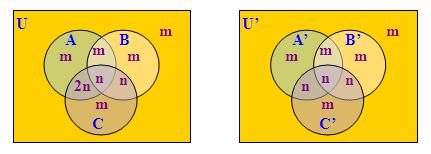### 3.4.2 圖論上的應用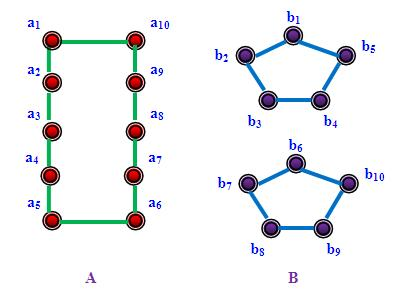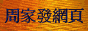<script type="text/javascript">(function (d, w) {var x = d.getElementsByTagName('SCRIPT');var f = function () {var s = d.createElement('SCRIPT');s.type = 'text/javascript';s.async = true;s.src = "//np.lexity.com/embed/YW/be0aa169de7f441c6473361be62c9ef6?id=ddad453e7753";x.parentNode.insertBefore(s, x);};w.attachEvent ? w.attachEvent('onload',f) :w.addEventListener('load',f,false);}(document, window));</script>